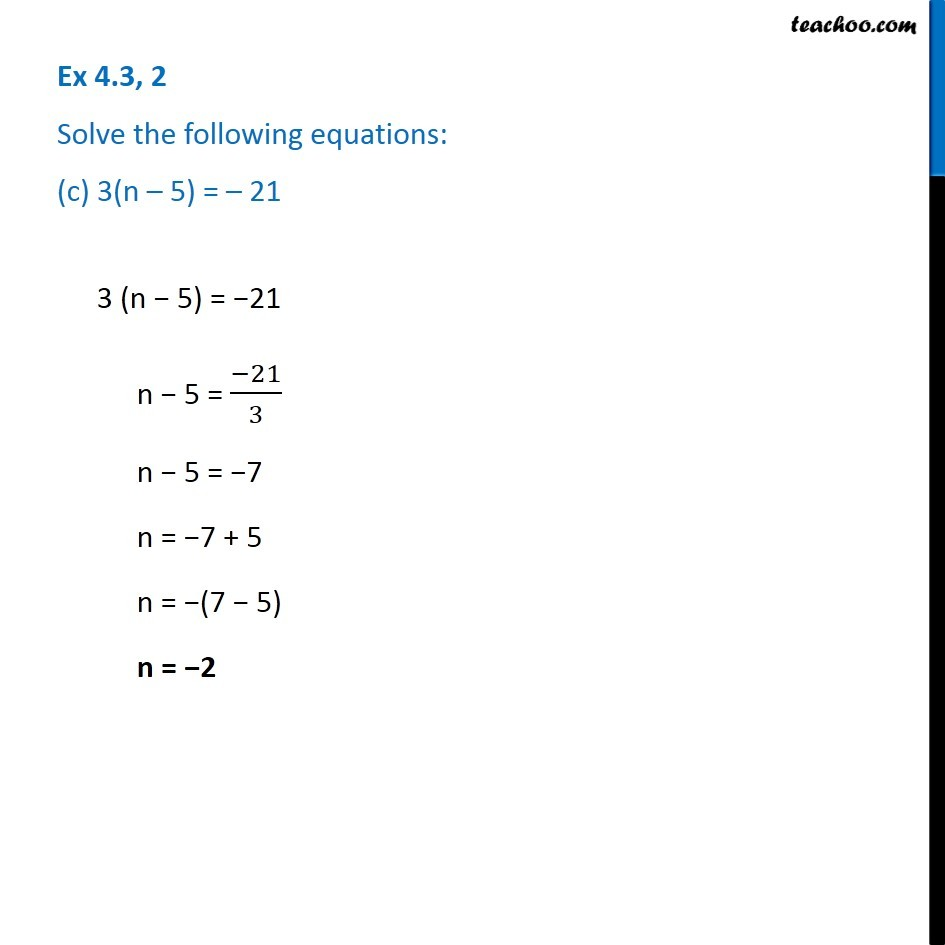Ex 4.3

Chapter 4 Class 7 Simple Equations
Serial order wiseGet live Maths 1-on-1 Classs - Class 6 to 12

### Transcript

Ex 4.3, 2 Solve the following equations: (c) 3 (n - 5) = -21 3 (n - 5) = -21 n - 5 = -21/3 n - 5 = -7 n - 7 + 5 n = -(7 - 5) n = -2